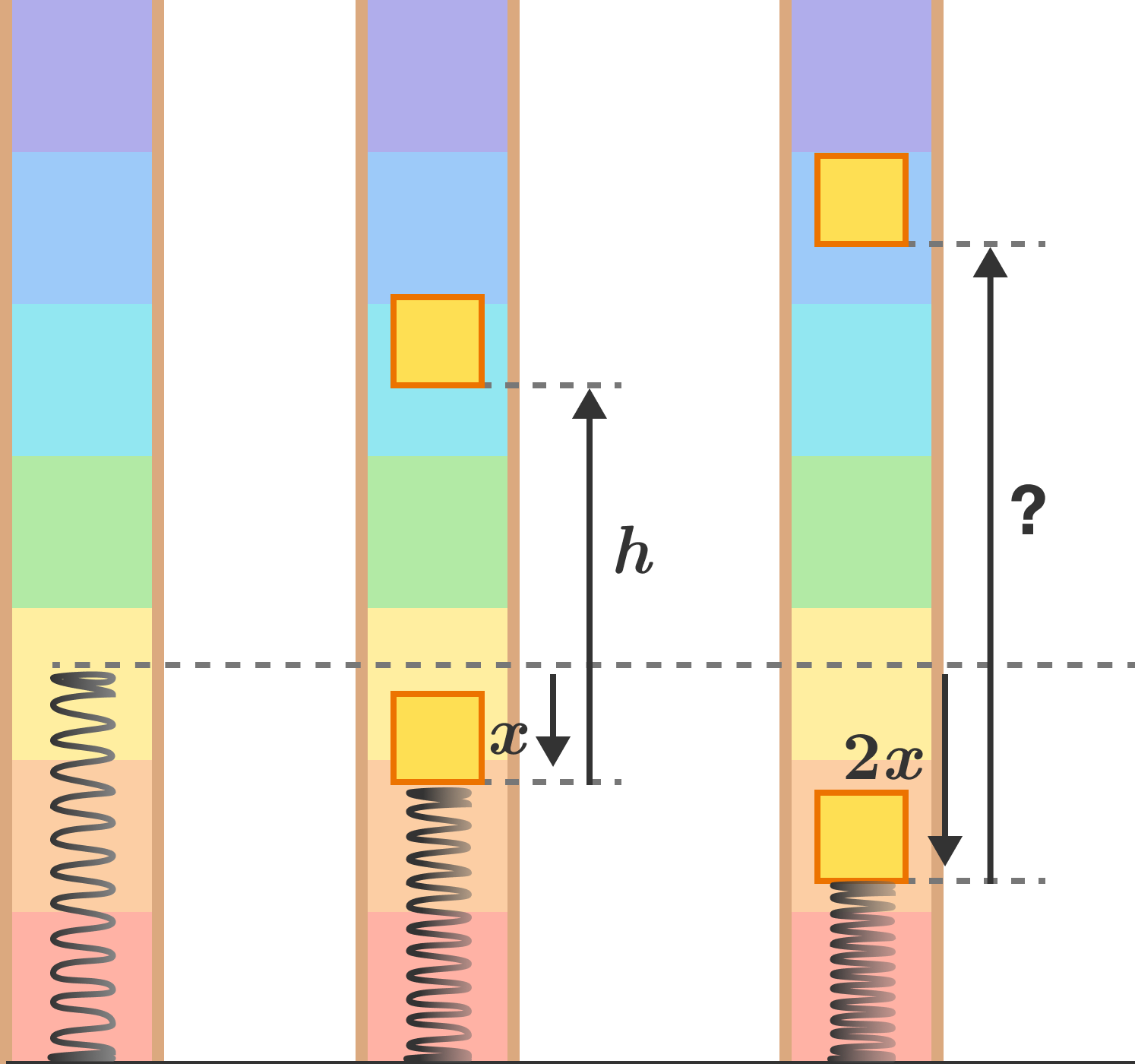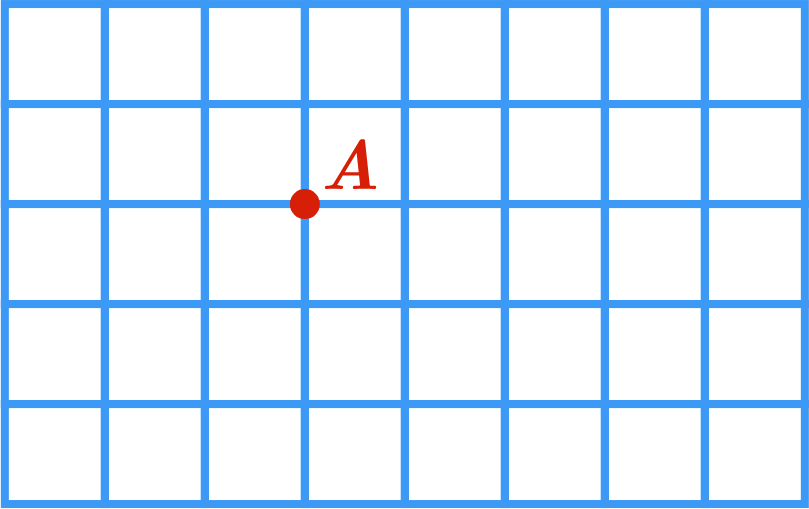Problems of the Week

Contribute a problem

2018-09-10 Intermediate

Creed plays a carnival game where he uses a spring to launch a block of wood. If he compresses the spring by $x$ from its natural length and releases it, the block rises to a height of $h$ from the point of release.

If he compresses the spring down the block by $2x$ from its natural length, how high will the block go up from the point of release?Is there an integer $n>1$ such that $n!$ is a perfect square?

A rectangle of a variable size is chosen at random from the $8 \times 5$ grid.

What is the probability the chosen rectangle has a vertex at point ${\color{#D61F06}A}?$You are playing a game with 2018 bins arranged in a circle. At the start, each bin has a white or black ball inside, with the two colors alternating between adjacent bins all throughout the circle.

The rules are as follows:

• Each turn, you select two balls. Any white ball you select moves clockwise to the next bin. Any black ball you select moves counterclockwise to the next bin.
• You may repeat this process as many times as you like.
• The two balls selected can come from the same bin at later stages when a single bin can have multiple balls.
• You win the game if all 2018 balls are in the same bin after a turn.

Is it possible to win this game?

A triangle’s perimeter and area have the same integer value.

What is the smallest possible area of the triangle?

×